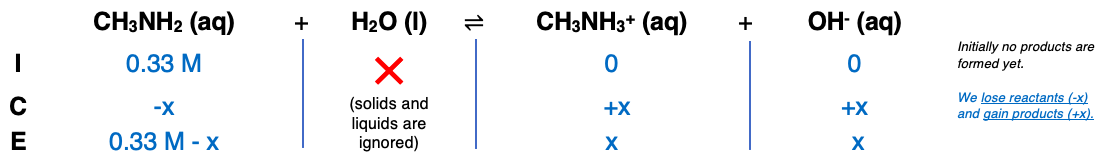# Problem: Find [OH−] of a 0.33 M methylamine (CH3NH2) solution. (Methylamine has a Kb value of 4.4×10−4.) Express the concentration in moles per liter to two significant figures.Find the pH of the 0.33 M methylamine (CH3NH2) solution. Express the pH of the solution to two decimal places

###### FREE Expert Solution

Step 1Step 2

→ remove (-x)

92% (277 ratings)###### Problem Details

Find [OH] of a 0.33 M methylamine (CH3NH2) solution. (Methylamine has a Kb value of 4.4×10−4.) Express the concentration in moles per liter to two significant figures.

Find the pH of the 0.33 M methylamine (CH3NH2) solution. Express the pH of the solution to two decimal places